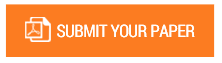The Linear Equation for 2D Graphs

Authors : Rumani Dey

Volume/Issue : Volume 5 - 2020, Issue 6 - June

Scribd : https://bit.ly/3095JbW

DOI : 10.38124/IJISRT20JUN1015

What is y=mx+c? What is one dimensional? If a thing like fish/bird while in motion in water/air cannot turn left, right and backward. It can only move forward in a medium or fly in air. When I try to determine its motion, I see it as one dimensional. It is not necessary that the bird/fish is moving in a straight line. It is the sheer concentration of the medium the fish /bird is traversing in. So, the behavior of a one dimensional motion maybe a function of the medium with a concentration/penetration quotient of the medium based on the weight of the fish/bird which penetrates the medium and the effect of gravity on it. We can depict a one dimensional motion as, One Dimensional motion=f(weight)* f(penetration quotient)*f(Force exerted by the fish/bird while it is penetrating the medium) What is one dimensional coordinate? We can say that it is a vector (V) which moves in any direction but not necessarily a straight line . If “x” is a one dimensional coordinate value, it is very difficult to find the value of “x” . If suppose the path traversed by “x ” is not a definite symmetrical pattern but an ambiguous pattern like below: Fig 1 Then we do not have a numerical value for “x”. It is not measurable through a scale. We cannot find the value of “x”. In this case the only way “x” can be measured is through a straight line if “x” is moving in one direction only. A question arises whether time is moving in a circular pattern or in a straight line .We measure it as a distance/displacement and we have deemed such a scale of measurement as one dimensional. But I want to measure the quantity the fish/bird had travelled in one minute. We might get some value by defining the area it had traversed in one minute which is a two dimensional quantity.

#### CALL FOR PAPERS

Paper Submission Last Date
31 - May - 2023